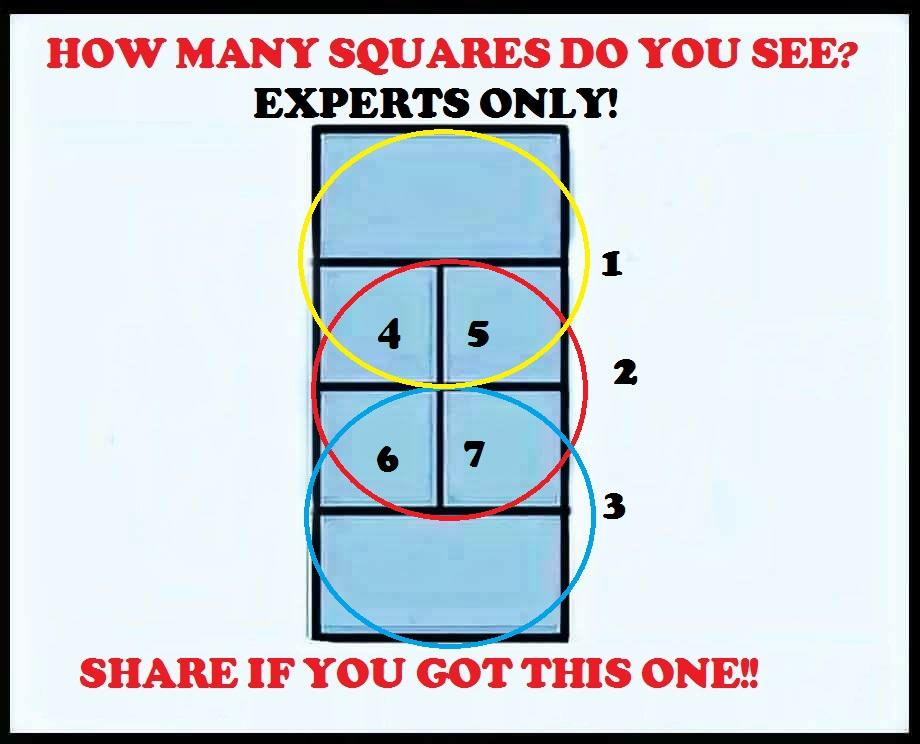# How Many Squares Puzzle

Can you count all squares in this pic? 95% people fail to answer it correctly. Let us see if you are one of those 5% who answered it correctly.

Use comment section and let us know how many squares you could find. You can also match your answer with the one we have published below.

Also Try: If air is called green – puzzle

Answer: There are 7 Squares

Explanation:Like our Facebook Page: Puzzles, Brainteasers and Fun

Search Keywords:

Count number of squares, squares counting puzzles, count squares puzzles, how many squares riddle, count squares in picture, how many squares puzzle answer